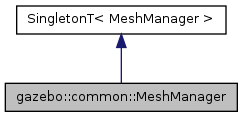##gazebo::common::MeshManager Class Reference

Maintains and manages all meshes. More...

`#include <common/common.hh>`

Inheritance diagram for gazebo::common::MeshManager:[legend]

## Public Member Functions

Add a mesh to the manager.
void CreateBox (const std::string &_name, const math::Vector3 &_sides, const math::Vector2d &_uvCoords)
Create a Box mesh.
void CreateCamera (const std::string &_name, float _scale)
Create a Camera mesh.
void CreateCone (const std::string &_name, float _radius, float _height, int _rings, int _segments)
Create a cone mesh.
void CreateCylinder (const std::string &_name, float _radius, float _height, int _rings, int _segments)
Create a cylinder mesh.
void CreatePlane (const std::string &_name, const math::Plane &_plane, const math::Vector2d &_segments, const math::Vector2d &_uvTile)
Create mesh for a plane.
void CreatePlane (const std::string &_name, const math::Vector3 &_normal, double _d, const math::Vector2d &_size, const math::Vector2d &_segments, const math::Vector2d &_uvTile)
Create mesh for a plane.
void CreateSphere (const std::string &_name, float _radius, int _rings, int _segments)
Create a sphere mesh.
void CreateTube (const std::string &_name, float _innerRadius, float _outterRadius, float _height, int _rings, int _segments)
Create a tube mesh.
void GenSphericalTexCoord (const Mesh *_mesh, math::Vector3 _center)
generate spherical texture coordinates
const MeshGetMesh (const std::string &_name) const
Get a mesh by name.
void GetMeshAABB (const Mesh *_mesh, math::Vector3 &_center, math::Vector3 &_min_xyz, math::Vector3 &_max_xyz)
Get mesh aabb and center.
bool HasMesh (const std::string &_name) const
Return true if the mesh exists.
bool IsValidFilename (const std::string &_filename)
Checks a path extension against the list of valid extensions.
Load a mesh from a file.Static Public Member Functions inherited from SingletonT< T >
static T * Instance ()
Get an instance of the singleton.Protected Member Functions inherited from SingletonT< T >
SingletonT ()
Constructor.
virtual ~SingletonT ()
Destructor.

## Detailed Description

Maintains and manages all meshes.

## Member Function Documentation

 void gazebo::common::MeshManager::AddMesh ( Mesh * _mesh )

Add a mesh to the manager.

This MeshManager takes ownership of the mesh and will destroy it. See ~MeshManager.

Parameters
 void gazebo::common::MeshManager::CreateBox ( const std::string & _name, const math::Vector3 & _sides, const math::Vector2d & _uvCoords )

Create a Box mesh.

Parameters
 [in] _name the name of the new mesh [in] _sides the x y x dimentions of eah side in meter [in] _uvCoords the texture coordinates
 void gazebo::common::MeshManager::CreateCamera ( const std::string & _name, float _scale )

Create a Camera mesh.

Parameters
 [in] _name name of the new mesh [in] _scale scaling factor for the camera
 void gazebo::common::MeshManager::CreateCone ( const std::string & _name, float _radius, float _height, int _rings, int _segments )

Create a cone mesh.

Parameters
 [in] _name the name of the new mesh [in] _radius the radius of the cylinder in the x y plane [in] _height the height along z [in] _rings the number of circles along the height [in] _segments the number of segment per circle
 void gazebo::common::MeshManager::CreateCylinder ( const std::string & _name, float _radius, float _height, int _rings, int _segments )

Create a cylinder mesh.

Parameters
 [in] _name the name of the new mesh [in] _radius the radius of the cylinder in the x y plane [in] _height the height along z [in] _rings the number of circles along the height [in] _segments the number of segment per circle
 void gazebo::common::MeshManager::CreatePlane ( const std::string & _name, const math::Plane & _plane, const math::Vector2d & _segments, const math::Vector2d & _uvTile )

Create mesh for a plane.

Parameters
 [in] _name [in] _plane plane parameters [in] _segments number of segments in x and y [in] _uvTile the texture tile size in x and y
 void gazebo::common::MeshManager::CreatePlane ( const std::string & _name, const math::Vector3 & _normal, double _d, const math::Vector2d & _size, const math::Vector2d & _segments, const math::Vector2d & _uvTile )

Create mesh for a plane.

Parameters
 [in] _name the name of the new mesh [in] _normal the normal to the plane [in] _d distance from the origin along normal [in] _size the size of the plane in x and y [in] _segments the number of segments in x and y [in] _uvTile the texture tile size in x and y
 void gazebo::common::MeshManager::CreateSphere ( const std::string & _name, float _radius, int _rings, int _segments )

Create a sphere mesh.

Parameters
 [in] _name the name of the mesh [in] _radius radius of the sphere in meter [in] _rings number of circles on th y axis [in] _segments number of segment per circle
 void gazebo::common::MeshManager::CreateTube ( const std::string & _name, float _innerRadius, float _outterRadius, float _height, int _rings, int _segments )

Create a tube mesh.

Generates rings inside and outside the cylinder Needs at least two rings and 3 segments

Parameters
 [in] _name the name of the new mesh [in] _innerRadius the inner radius of the tube in the x y plane [in] _outterRadius the outer radius of the tube in the x y plane [in] _height the height along z [in] _rings the number of circles along the height [in] _segments the number of segment per circle
 void gazebo::common::MeshManager::GenSphericalTexCoord ( const Mesh * _mesh, math::Vector3 _center )

generate spherical texture coordinates

 const Mesh* gazebo::common::MeshManager::GetMesh ( const std::string & _name ) const

Get a mesh by name.

Parameters
 [in] _name the name of the mesh to look for
Returns
 void gazebo::common::MeshManager::GetMeshAABB ( const Mesh * _mesh, math::Vector3 & _center, math::Vector3 & _min_xyz, math::Vector3 & _max_xyz )

Get mesh aabb and center.

Parameters
 [in] _mesh the mesh [out] _center the AAB center position [out] _min_xyz the bounding box minimum [out] _max_xyz the bounding box maximum
 bool gazebo::common::MeshManager::HasMesh ( const std::string & _name ) const

Return true if the mesh exists.

Parameters
 [in] _name the name of the mesh
 bool gazebo::common::MeshManager::IsValidFilename ( const std::string & _filename )

Checks a path extension against the list of valid extensions.

Returns
true if the file extension is loadable
 const Mesh* gazebo::common::MeshManager::Load ( const std::string & _filename )

Load a mesh from a file.

Parameters
 [in] _filename the path to the mesh
Returns
a pointer to the created mesh

The documentation for this class was generated from the following file: